Orginal Article

# ONE-DIMENSIONAL QUASI-STATIC CONSOLIDATION MODEL CONSIDERING INERTIA OF FLUID PHASE

School of Civil Engineering, Beijing Jiaotong University, Beijing 100044,China

Abstract

Terzaghi’s one-dimensional classic consolidation theory ignores inertia of pore water in saturated soils, and has outstanding differences in its derivation and formulation during various publication periods. This leads to a strange phenomenon that considerable misunderstandings and confusion of it still prevail in the current literature. Within the previous framework of large strain dynamic consolidation theory, a one-dimensional infinitesimal strain consolidation wave model is obtained to consider the effect of inertia of pore water with necessary simplification and hypotheses. The present consolidation wave model is characterized by velocity dispersion and dissipative attenuation. The method of separation of variables is used to obtain an analytical solution for a consolidation wave model under the condition of one-way drainage and instantaneous loading. A numerical case study shows that behaviors of consolidation wave are actually controlled by the value of a dimensionless number $Dc$. For cases of higher values of $Dc$, jump and fluctuation of dimensionless excess pore water pressure between positive and negative values are prone to occur, in contrast with the cases of lower values of $Dc$ that result into special phenomena including the Mandel-Cryer effect observed in laboratory tests. The inherent ambiguities about Terzaghi’s classic theory models proposed in the early stage and the later stage respectively are investigated to draw a conclusion that the early Terzaghi’s (1923,1925) consolidation model can be interpreted as a large strain model with respect to the general soil coordinates contrasted with an infinitesimal strain model with respect to the solid-phase volume coordinates. Accordingly, the present consolidation wave model can be extended to various formulations based on the corresponding coordinate properties. Consolidation wave theory is of significance to probe into an innovative uncertainty principle which shows that for scale model testing, the observed consolidation wave response of undisturbed soil samples cannot equal the response of the same soil in practical conditions. Therefore, it is advisable to pay attention to the size effect of soil samples in consolidation study from the perspective of microscopic soil mechanics. The theoretical precision of evaluated excess pore water pressure by classic Terzaghi’s consolidation model varies with the value of dimensionless quantity $Dc$, which is a vital parameter in the proposed consolidation wave theory.

Keywords： consolidation wave ; inertia of pore water ; consolidation ; Terzaghi’s one-dimensional consolidation theory; ; Mandel-Cryer effect ; jump phenomenon

0

Ding Zhouxiang. ONE-DIMENSIONAL QUASI-STATIC CONSOLIDATION MODEL CONSIDERING INERTIA OF FLUID PHASE[J]. Acta Mechanica Sinica, 2018, 50(4): 908-928 https://doi.org/10.6052/0459-1879-18-053

## 引言

$c∂2w∂z2=∂w∂t(1)$

$krav∂2u∂z2=∂u∂t(2)$

$krγwav∂2u∂z2=∂u∂t(3)$

$k1+eγwav∂2u∂a2=∂u∂t(4)$

Znidarcic和Schiffman在针对文献讨论稿 $$答复中,补充了控制方程 $$

$∂∂zkγw1+e∂u∂z+∂e∂t=0(5)$

$∂∂kr∂u/γw∂z=av∂u∂t(6)$

$∂u∂t=cvc∂2u∂z2(7)$

$cvc=k/γwmvc=k1+e0/γwavc(8)$

$cv∂2u'∂z2=∂u'∂t(9)$

$cv=kv/γwmv=kv1+e/γwav(10)$

## 1 Terzaghi (1943)固结理论模型

### 1.1 基本假定

Terzaghi采用的基本假定如下 $$：(1)土体孔隙完全充满水;(2)土体中水和固相成分均不可压缩;(3)达西定律严格成立;(4)渗透系数 $k$是常数;(5)固结时滞完全出于土体低渗透性. 补充假定有：(1)黏土侧向受限;(2)对固结过程每个阶段,任意水平剖面内各点总正应力和有效正应力相等;(3)有效应力从初始值 $p̅0$增加到最终值 $p̅$将使黏土孔隙比由初始值 $e0$减小到最终值 $e$;(4)压缩系数 $avc$在有效应力增大范围内是常数.

### 1.2 固结过程微分方程

1.2.1 有效应力方程

$p̅0=p0-uw(11)$

2cm $u+p̅-p̅0=p1(12)$

$∂u∂t=-∂p̅∂t(13)$

1.2.2 土体骨架本构关系

$n0-n=mvcp̅-p̅0(14)$

$∂p̅∂t=-1mvc∂n∂t(15)$

$∂n∂t=mvc∂u∂t(16)$

Terzaghi将式(16)应用于厚度 $dz$的薄层土体

$-∂n∂tdz=-mvc∂u∂tdz(17)$

1.2.3 连续性方程

$∂v∂zdz=-∂n∂tdz(18)$

1.2.4 土体液相本构方程：Darcy渗流定律

Terzaghi使用Darcy定律,将考察点在时刻 $t$

$v=ik(19)$

13mm $i=-∂h/∂z=-1/γw∂u/∂z(20)$

1.2.5 固结控制方程的导出

### 1.3 对Terzaghi(1943)模型的再认识

$cvcz,t=kt/γwmvcz(21)$

## 2 一维小变形固结波模型的建立

### 2.1 力学基础

$vsi,i=-qi,i(22)$

$τji,j+ρfi=ρv̇si-vsiρfqj,j+ρfvsj,jqi+2mm]Dρfqi/Dt(23)$

$∂τ/∂x+ρf=ρfq̇(24)$

$∂vs/∂x=-∂q/∂x(25)$

### 2.2 一维小变形固结波的基础方程

2cm $∂σ/∂z+ρf=ρf∂q/∂t(26)$

2cm $∂vs/∂z=-∂q/∂z(27)$

### 2.3 固相速度场散度及其转换所需基本方程

2.3.1 固相速度场散度的等价变换

$∂vs∂z=∂∂z∂us∂t=∂∂t∂us∂z=∂εs∂t(28)$

2.3.2 土体小应变时间变化率及骨架本构的引入

$εs=e-e0/1+e0(29)$

$∂εs∂t=11+e0∂e∂t=11+e0dedσ'∂σ'∂t=2mm]-av1+e0∂σ'∂t(30)$

2.3.3 有效应力时间变化率及有效应力方程的引入

$σ'=σ-uw(31)$

$∂σ'/∂t=∂σ/∂t-∂uw/∂t(32)$

2.3.4 总应力时间变化率及准静力平衡方程、土水势方程和Darcy定律的引入

$σ=∫zGSz‍ρf∂q/∂tdz+pGSt(33)$

$∂σ∂t=∂∂t∫zGSz‍ρf∂q/∂tdz+∂pGSt∂t(34)$

$∂∂t∫zGSz‍ρf∂q/∂tdz=∫zGSz‍∂∂tρf∂q/∂tdz(35)$

$ψ=γfz-zdp+uw=γfz-zdp+γfzwt-z+ue(36)$

$ψ=γfzwt-zdp+ue(37)$

$q=-kγf∂ψ∂z=-kγf∂ue∂z(38)$

$∫zGSz‍∂∂tρf∂q/∂tdz=-ρfkγf∫zGSz‍∂2∂t2∂ue∂zdz(39)$

$∫zGSz‍∂2∂t2∂ue∂zdz=∂2ue∂t2z-∂2ue∂t2zGS(40)$

$∂2ue/∂t2zGS=0(41)$

$∂σ∂t=-kg∂2ue∂t2+∂pGSt∂t(42)$

2.3.5 孔压变化率及孔压方程的引入

$uw=γfzwt-z+ue(43)$

$∂zwt-z/∂t=0$条件下,孔压和超静孔压的时间变化率满足

$∂uw/∂t=∂ue/∂t(44)$

$∂vs∂z=av1+e0kg∂2ue∂t2-∂pGSt∂t+∂ue∂t(45)$

### 2.4 液相速度场散度及其转换所需的基本方程

$-∂q∂z=-∂∂z-kγf∂ue∂z=kγf∂2ue∂z2(46)$

### 2.5 一维小变形固结波的控制方程

$ca∂2ue∂t2-∂pGSt∂t+∂ue∂t=cv∂2ue∂z2(47)$

12mm $ca=k/g(48)$

12mm $cv=k1+e0/γfav(49)$

$ca∂2ue∂t2+∂ue∂t=cv∂2ue∂z2(50)$

## 3 一维小变形固结波基本特征

### 3.1 固结波的频散关系

$uez,t=eikzz-ωt(51)$

$0,将式(51)代入 式(50),得

$caω2+iω-cvkz2=0(52)$

$ω=-i±4cacvkz2-12ca=±4cacvkz2-12ca-i12ca(53)$时,式(52)的解答为

$kz<1/2cacv$

$Imω„0$

$kz<1/2cacv$

$Imω$

### 3.2 固结波的波动方程形式

$ct$

$vp2=ct2-γ24kz2

15mm $∂uez,t∂z=0,z=0(61)$

15mm $uez,t=0,z=H(62)$

## 4 固结波一般定解问题及解析解

### 4.1 一维小变形固结波定解问题

10mm $fz$

10mm $gz$

$Dc2∂2Φ∂Tv2+∂Φ∂Tv=∂2Φ∂ξ2(65)$

$Φ=ue/p,p=maxfz(66)$

### 4.2 固结波定解问题的无量纲化

$Tv=cvt/H2(68)$

15mm $Dc2=cacv/H2(69)$

15mm $∂Φ/∂ξ=0,ξ=0(70)$

15mm $Φξ,Tv=0,ξ=1(71)$

15mm $Φξ,Tv=fξ,0„ξ<1,Tv=0(72)$

15mm $∂Φξ,Tv/∂Tv=gξ,0„ξ<1,Tv=0(73)$

15mm $Φξ,Tv=ΞξTTv(74)$

$Ξ''ξTTv=Dc2ΞξT''Tv+ΞξT'Tv(75)$

$ΞξTTv$

### 4.3 固结波定解问题的分离变量法解答

4.3.1 分离变量

$-λ$

$Ξ''ξΞξ=Dc2T''TvTTv+T'TvTTv=-λ(76)$

$φξ,Tv$

$Ξ'ξ=0,ξ=0,Tv=Tv(79)$

$Ξξ=0,ξ=1,Tv=Tv(80)$

$ΞξT0=fξ,0„ξ<1(81)$代入边界条件式(70)和式(71),得

$ΞξT'0=gξ,0„ξ<1(82)$

$λ„0$

$Ξξ$

$λ>0$

4.3.2 求解本征值问题

$Ξnξ=cos2n+1ξ/2,n=0,1,2,⋯(85)$

$n=0,1,2,⋯$

$α=1/2Dc2(86)$

4.3.3 一般解

$βn=Δ/2Dc2,n=0,1,2,⋯(87)$, $r1,2=-α±Δ/2Dc2(88)$,以及

$Dc2$

$Δ≠0$

$Δ>0$

$n>1/Dc-1/2,n=0,1,2,⋯(91)$

$Tnt=e-αTvAncosβnTv+BnsinβnTv(92)$

(3) $FDc=[1/(Dc)-1]/2$,即

$FDc≠0$

$N$

$cos2n+12ξ+$

$∑n=N+1∞‍e-αTvAncosβnTv+BnsinβnTv⋅$

$cos2n+12ξ(94)$

$1.7977×10+308$

$e-αTvAncoshβnTv+BnsinhβnTv=2mm]12Ane-α+βnTv+e-α-βnTv+2mm]12Bne-α+βnTv-e-α-βnTv(95)$

$An$

$An,Bn$

4.3.4 初始条件的引入及系数 $n=0,1,2,⋯$, $∑n=0∞‍Ancos[(2n+1)ξ/2]=f(ξ)(96)$确定

$gξ$

$(-1,0]$

$fz=p1$

$$

(98)和式(99),得到

$u(z,t)=4p1∑k=0,1,⋯∞‍-1k2k+1e-2k+122cvt4H2⋅2mm]cos2k+1z2H(101)$

## 5 经典算例的Terzaghi解和固结波解

### 5.1 Terzaghi固结解答及其无量纲化

$$

$z'=H$

$ΦTξ,Tv=4∑k=0,1,⋯∞‍-1k2k+1e-2k+122Tv4cos2k+1ξ2(105)$

$gξ=0,0„ξ<1(107)$

$An=4/π-1n/2n+1,n=0,1,2,⋯(108)$

### 5.2 经典算例固结波理论解

12mm $Bn=4α/π-1n/2n+1/βn,n=0,1,2,⋯(109)$

12mm $Φξ,Tv$

$Dc2$

$$

## 6 算例参数研究与对比分析

### 6.1 参数变化范围和计算条件

$N$

$Dc$数值或对应的 $N=1$值对固结波函数 $Dc$的变化规律有重要影响. 以下将重点针对 $Dc=0.1$值较大和较小两种典型情况,探讨固结波模型蕴含的阶跃效应和Mandel-Cryer效应,并与Terzaghi(1943)固结模型加以对比.

### 6.2 阶跃现象与波动效应

6.2.1 $N=1$与一级阶跃

$Nud=3×105$较大的情况,首先不妨以 $ξ=0$为例：在固结波级数解答式(94)的数学结构中, $ξ=0.5$. 这意味着固结波解中仅有2项涉及过阻尼机理贡献,其余则为欠阻尼机理贡献. 兼顾精度和效率起见,欠阻尼级数和中,取 $ξ=0.9$. 考察不透水边界点 $Φ$、中点 $Dc=0.1$和距离排水边界为0.1的点 $Φ$等3个代表点,其时程曲线如图1所示.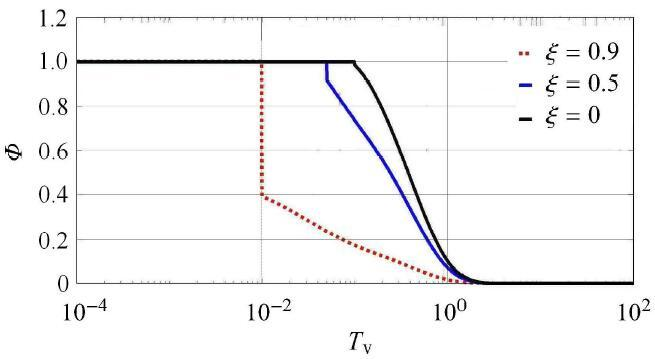Fig. 1   Consolidation wave $Φ(0.9,0.00998)≈1.00$ and time curve for 3 points ($Φ0.9,0.01005≈0.39$

6.2.2 $γΦ=1/Dc2$与多级阶跃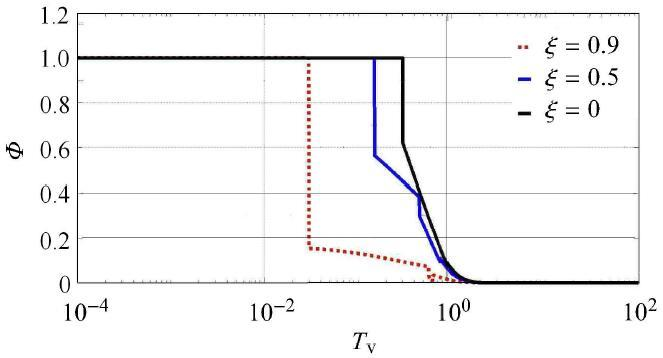Fig. 2   Consolidation wave $Dc>1/$ and time curve for 3 points ($N=-1$

6.2.3 $Φ$与波动中的负压

$Dc=0.5$时,恒有$10.0$,此时固结波函数$Φ$将不受过阻尼项任何影响,而呈现完全欠阻尼波 动特点. 不妨以$Dc=0.5$$Φ$为例继续计算,结果分别如图3图4所示.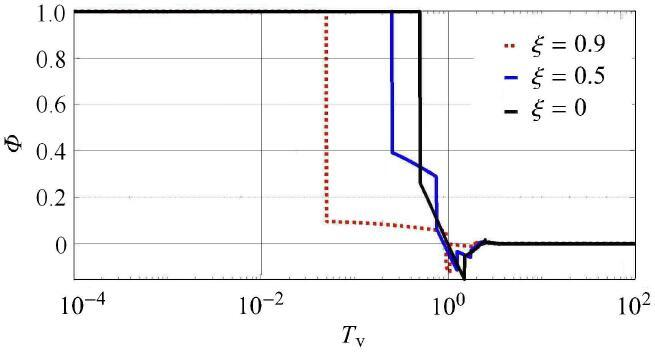Fig. 3   Consolidation wave $Dc=10.0$ and time curve for 3 points ($Φ$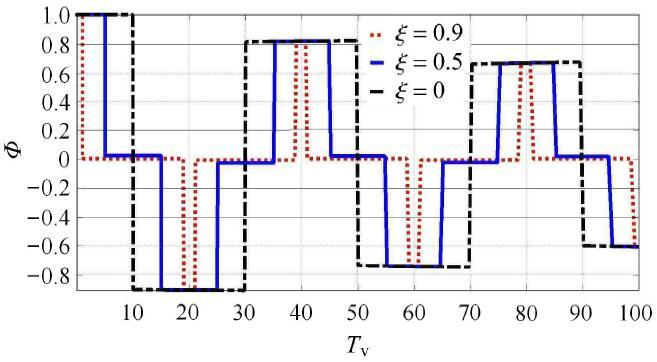Fig. 4   Consolidation wave $Dc$ and time curve for 3 points ($Tv$

### 6.3 固结波与Terzaghi(1943)固结理论对比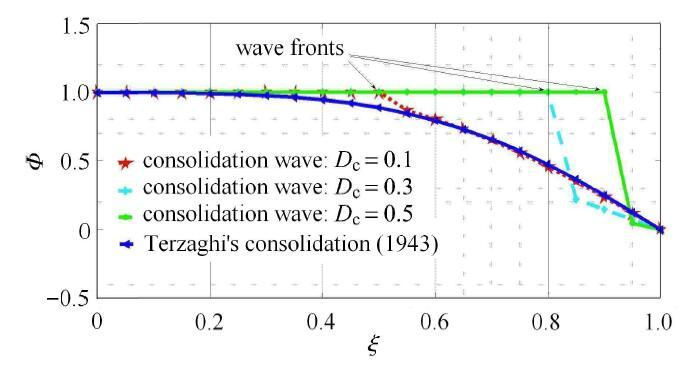Fig. 5   Spatial distribution of consolidation wave $Dc$ for various $Φ$ ($Tv=0.2003)$)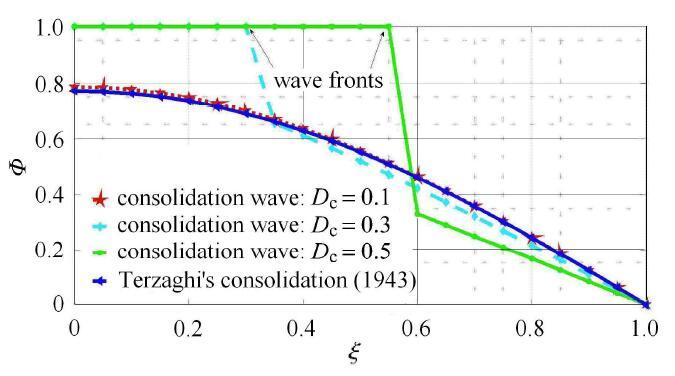Fig. 6   Spatial distribution of consolidation wave $Tv=0.0499$ for various $ξ$ ($Φ$)

$ξ=0$发展到0.203 3时,在图6$Φ$的固结波波前早已通过不透水边界 $Φ$,故 $Dc$$ξ=0$值有明显下降,并且各点 $ξ=0.5$值总体上 与Terzaghi理论曲线相符. 其他2种 $Dc$值对应的波前已更靠近但仍未达到不排水面,所以仍存在阶跃.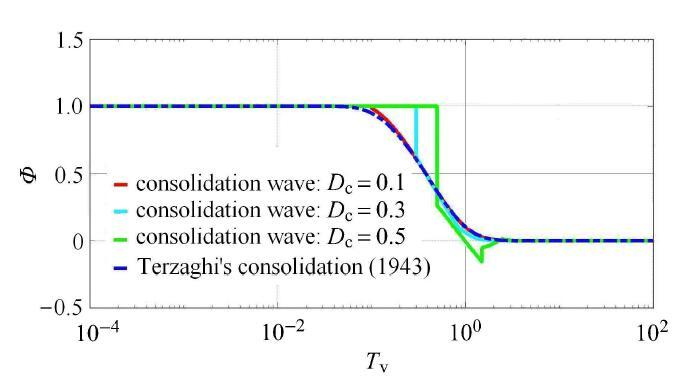Fig. 7   Consolidation wave $Dc$ and time curve for various $Φ$ ($ξ=0.5)$)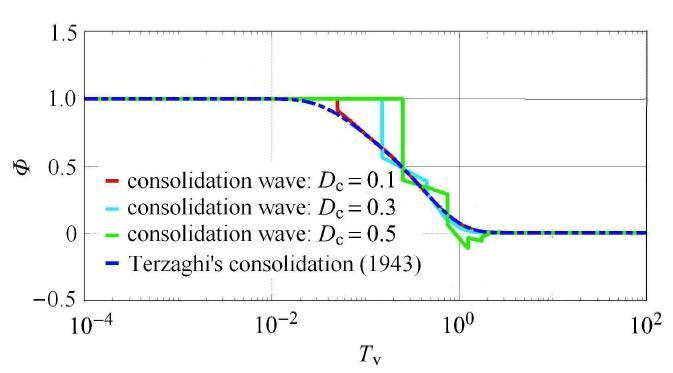Fig. 8   Consolidation wave $Φ$ and time curve for various $Dc=0.1)$ ($Φ$)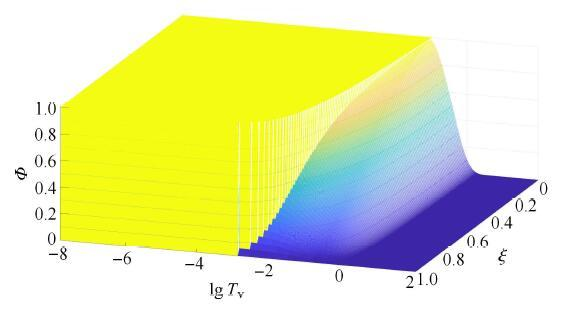Fig. 9   Spatial and time distribution of excess pore water pressure $Φ$ by consolidation wave model($$)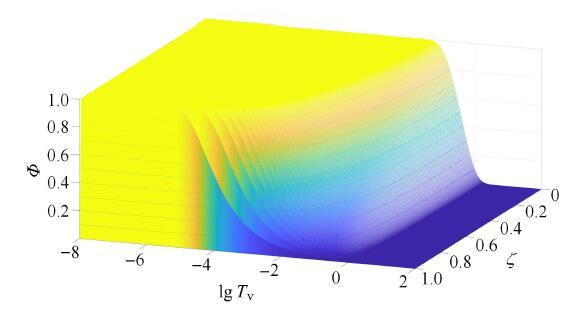Fig. 10   Spatial and time distribution of excess pore water pressure $t1$ by Terzaghi’s model (1943)

### 6.4 Mandel-Cryer效应的新机理

6.4.1 Mandel-Cryer效应机理经典诠释

Mandel-Cryer效应是指在一定条件下,固结初期部分土体中孔隙水压力不仅不消散而且呈上升的现象 $rB$. 钱家欢和殷宗泽 $rB$对均布压力作用的土球径向向外排水固结问题,提出Mandel-Cryer效应的一种机理解释：设在固结初期某一时间 $rB$,排水边界周边处孔压下降仅波及到半径 $rB$之外, $$以内孔隙水尚未排出;此时 $$以外土体固结过程将产生收缩,由此引起内部总应力随时间而提高;在 $∂pGSt/∂t=0$以内土体不固结前提下,这种外壳收缩引起的总应力增大只能通过内部孔压提高来承担. 龚晓南 $∂σ/∂t=-[kt/g]∂2ue/∂t2(111)$也提出一种精辟解释：排水边界土体收缩变形对内部土体产生压缩力,由此引起超静孔压的提高量大于排水固结引起的下降量时,就产生Mandel-Cryer效应. 传统观点还认为,该效应仅是二维和三维固结中的现象,一维固结并不存在——因为没有总应力变化 $kt≠0$.

6.4.2 固结波模型对总应力时间变化率的考虑

$ue$

$∂2ue/∂t2$的固结过程,显然总应力时间变化率 $R=15$受超静孔压 $p=10$二阶时间变化率 $E=10$控制,而不再恒为零. 因此,一维固结同样具备Mandel-Cryer效应产生条件：总应力随时间变化.

6.4.3 Mandel-Cryer效应算例及其对称性和一维化

6.4.4 算例的固结波分析及Mandel-Cryer效应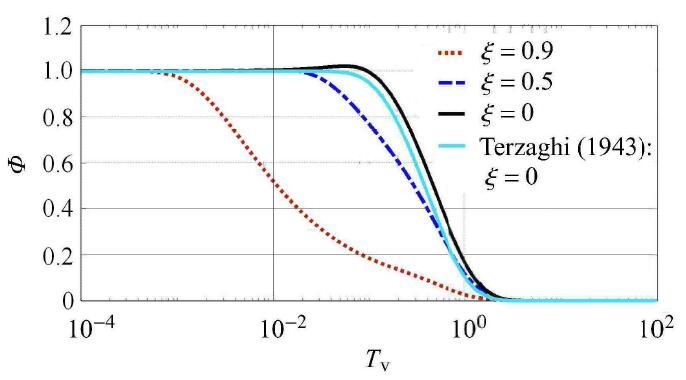Fig. 11   Case study of Mandel-Cryer effect ($ξ$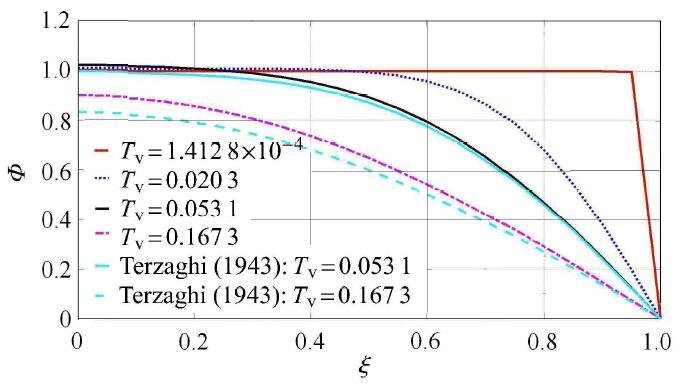Fig. 12   Spatial distribution of consolidation wave for Mandel-Cryer effect ($rB$)

6.4.5 算例结果简要对比

Mandel-Cryer效应中最大孔压的固结波预测值相对偏小的原因主要有：(1)不同分析中物理力学和几何参数的差异;(2)问题 维度差异;(3)土体体力的影响——固结波模型与Terzaghi理论都没考虑土体体力影响,但丁洲祥等 $ε-ε0=-ε0p2p=-ap(112)$认为土体体力具有 非保守属性,当变形较大时对结果影响显著;(4)材料和几何双线性化的影响;(5)黏性或次固结效应. 仇玉良和丁洲祥 $dε=-adp(113)$在一维小变形主、次固结耦合研究中发现,随着无量纲主、次固结耦合系数 $dq=-adp(114)$变化,单面排水不透水边界上无量纲超静孔压会在不同程度上超过外载荷,即出现类似Mandel效应现象——这与殷建 华等EVP模型预测的早期超静孔压异常升高的黏性本质是一致的. 但黏性效应造成初期超静孔压升高的同时降低了有效应力,固结过程中总应力不变,因此区别于本文固结波孔隙流体惯性机理. 在固结波模型中,如果进一步考虑黏性,那么固结初期超静孔压理论值会有所提高.

## 7 深入讨论和展望

### 7.1 Terzaghi固结理论的内在矛盾及改进历史

7.1.1 Terzaghi(1925)固结理论的基本方程

Terzaghi(1925)在推导其固结模型时,先后使用了如下方程. 固相本构方程

$ω=p1-p(115)$

$i=Qk=-dωdz(116)$

$dQdz=adpdt=-adωdt(117)$

$c∂2p∂z2=∂p∂t(118)$

$c=k/a$

$$

$$

7.1.2 Terzaghi(1925)模型的内在矛盾

Terzaghi(1925)模型 $a$是否是一种线性化大应变模型 $z$?笔者认为,问题核心在于连续性方程式(117)的解读方式. 当坐标 $a$为普通坐标且系数 $a$为压缩系数含义时,式(117)在力学意义上不成立. 如果在坐标 $~$为普通坐标条件下保证式(117)的正确性,则系数 $mvc$的含义应当是体积压缩系数. 但是,系数 $$已经在方程式(112) $$式(114)中被赋予压缩系数的含义,这样就产生了内在矛盾.

7.1.3 Terzaghi(1943)模型中的努力： $$的引入

Terzaghi $$和Terzaghi和Jelinek $~$先后不断努力解决上述矛盾. 首先,在固相本构关系的表征方面,Terzaghi $~$在与Terzaghi $mvc$基本一致的孔隙比--有效应力关系方程(文献式(1) $$式(3))后面,没有说明原因情况下,以应变--有效应力关系形式,补充了表示相同固相本构规律 的方程(文献式(4) $mvc$式(6)),其中涉及新的体积减小系数 $$(文献式(5)). Terzaghi $a$在连续性条件中以 $z$替代Terzaghi $n$模型式(117)中的系数 $$. 于是,上述逻辑矛盾暂时得到解决：坐标 $$是普通坐标,且连续性方程正确.

7.1.4 Terzaghi(1943)模型中的努力：新矛盾即 $e0$歧义

$n$

$n01$

Terzaghi把 $n$理解为黏土的孔隙体积. 显然,如果要保持前后一致,式(120)中 $Δs$应当为 $n$——概念 $$的内涵产生歧义.

7.1.5 Terzaghi(1954)模型的努力： $Δs$的引入

$Δs=ε-ε01+ε0=⋯=mvcp̅-p̅0=mvcΔp̅(123)$

$ε-ε0$

$$

Terzaghi理论的自我完善在力学上局限于本文连续性条件式(27)的范畴.

### 7.2 Terzaghi固结理论2种小变形固结系数的起源

Taylor $1+e$认为土体微元体积在固结过程中固相体积不变,据此求出单位土体微元体积时间变化率,相当于式(30)第1个等号右端变为

$1+e0$

Taylor $$使用的坐标是欧拉坐标,而非普通坐标,这区别于Terzaghi $dxdydz/1+e0$作法. 很多文献沿用Taylor上述推导思路 $1+e$. 例如,Terzaghi等 $1+e0$引入 $$为常数的假定,却又前后不一致地采用 $cv$替换 $$,得到式(10)所示与Taylor结果 $cv$相同的固结系数 $$. 再以同济大学土力学教材为例,其第2版 $$$cv$是式(10),而第3版 $$和第5版 $$$∂∂zkeγw1+edσ'de∂e∂z+∂e∂t=0(125)$则为式(8),但没有见到深入讨论.

### 7.3 Gibson理论框架内Terzaghi理论的变形属性

$ke/1+e$

$k=ke/γw1+e$

### 7.4 Tsytovich理论与Terzaghi理论的联系

7.4.1 Tsytovich (崔托维奇) (1951)的固结控制方程

Tsytovich $t$提出一种固结模型

$c$

$ε1$

7.4.2 Tsytovich (1951)理论的坐标属性和关键概念

$z$

Tsytovich (1951)还给出3点：(1)“土的引用渗透系数” $q=-k0∂H/∂z(131)$概念,以区别于渗透系数 $H$,即

$∂q/∂z=-∂ε/∂t(132)$

(2) Darcy定律的表述形式

$ε$

$ζ$

$$

7.4.3 Tsytovich理论与Terzaghi理论的关系

Terzaghi用过特殊土体厚度 $c$概念 $$,即

$k$

$$

$$

ASCE $$报道过固结系数定义由式(136)到式(10)的奇怪变化,但没有原因说明. Taylor $$与Znidarcic和Schiffman $z$等也先后注意到这个问题. Terzaghi $$认为,Terzaghi $z$理论中坐标 $$具有固相体积坐标属性,不同于后期Terzaghi $$理论中 $[3,47]$的普通坐标属性,这种差异导致不同的控制方程及固结系数. 然而,Terzaghi $[9,47]$较早时却阐明：Terzaghi $[51,54]$模型与早期Terzaghi $[3,47]$模型是一样的. 显然Terzaghi $$论述之间充满自相矛盾.

$z$

### 7.5 2种坐标背景及4种固结系数

$cζ=cGEH=ke1+e02γw1+e-de/dσ'(139)$

$cζT,1925=cGEH(140)$

$cζTs,1940=1+e/1+e0cGEH(141)$

$$

$cζ=cζT,1943$

$cζ$

### 7.6 固结波模型拓展与历史背景

7.6.1 固结波模型拓展

$cζT,1925$

$cζ(Ts,1940)$时,式(144)等价于式(50). 当式(144)中 $cζTaylor,1948$分别取 $[5,57]$, $$$$时,则在形式上得到固结波模型其他3种表述形式.

7.6.2 Fillunger(1936)固结理论

$-∂n∂t+∂1-nvs∂z=0(148)$

$Z=-vw-vsn0k'γw(149)$

$~$

$n0$

$k'$

7.6.3 Fillunger理论分析及与固结波模型对比

$∂vs/∂t+vs∂vs/∂z=v̇s$

$ρv̂̇=-∂σ/∂z(152)$

$ρ=ρw+ρs$

$q0=vw-vs$

$nq̇0=q̇$

$$

7.6.4 Biot动力固结理论与固结波模型对比

Biot动力固结理论 $$比Fillunger $$理论滞后约20年,但前者逐渐发展成为主流并仍是研究热点,如宋佳等 $[24-27]$和Xu等 $[62-63]$在体积波传播特征等方面取得新认识. 然而,Voyiadjis和Song $$认为：Biot模型不是从力学和热力学基本公理和原理发展得出,所以复杂难懂. 在Biot $u-p$成果基础上, Zienkiewicz等 $Dc$和Zienkiewicz $Dc2=cacv/H2=k2Es/(g2ρwH2)$提出一种简化的 $$模型,其中仅考虑固相加速度而忽略液相加速度,不同于本文固结波仅考虑液相惯性的情况.

### 7.7 固结波的土力学意义及学科交叉

7.7.1 缩尺固结试验的不确定性原则

7.7.2 尺度效应与微观土力学

7.7.3 固结波与学科交叉

## 8 结 语

(1)基于丁洲祥等大变形动力固结理论 $Dc$,经过简化提出一个一维小变形固结波动模型,即式(50)和式(65). 固结波具有频散和耗散特征,其极限相位速度满足式(60)和式(58).

(2)采用分离变量法,得到了单面排水和瞬时加载条件下无量纲固结波定解问题的解析解答,即式(94),可为理论应用分析和校核数 值解法的精度提供可靠的依据. 固结波解析解即式(94)数学结构反映了动力学中过阻尼( $Φ$)和欠阻尼( $Dc$)两种机理的贡献.

(3)单面排水和瞬时加载情况下固结波分析结果表明：当无量纲数 $a$达到某些较大值时,无量纲超静孔压 $a$可能出现阶跃和波动现象;而当 $Dc$为某些较小数值时,对应固结波响应结果可以解释Mandel-Cryer效应的部分机理. 超静孔压发展的本质是固结波传播.

(4) Terzaghi经典固结理论系统分析结果表明：(a) Terzaghi模型方程其实可以部分反映渗透性和压缩性变化. (b)早期Terzaghi固结模型与后期Terzaghi固结模型可能不同,而Terzaghi(1943)和Terzaghi(1960)的解释相互矛盾. (c)当坐标理解为普通土体坐标时,早期Terzaghi模型就成为等同于后期模型的小变形模型,但会出现系数{Invalid MML}兼作压缩系数和体积压缩系数的错误. (d)当坐标理解为固相体积坐标时,早期Terzaghi模型就是一种与后期模型不同的大变形模型,此时系数{Invalid MML}将仅表示压缩系数. (e)不同时期Terzaghi固结模型的固结系数涉及4种定义和2种坐标性质,可统一于式(138). (f)经大变形诠释的早期Terzaghi固结模型比后期小变形模型在理论上更先进.

(5)鉴于经典一维固结理论上述复杂背景,固结波模型在形式上可拓展至相应的4种表述结果.

(6)固结波模型具有丰富的内涵：常规缩尺固结试验在理论上存在物理力学参数与固结波响应两种因素之间的不确定性矛盾; 通过微观土力学视角的固结研究宜重视尺寸效应. 土力学中可能隐含一种量子效应.

(7)在一定{Invalid MML}范围内,抛物型经典一维固结理论结果在不同程度上近似于双曲型固结波模型结果. 未来固结波理论发展,可以逐步考虑非线性、固相惯性和多场多相耦合,同时加强试验研究.

The authors have declared that no competing interests exist.

## 参考文献 原文顺序 文献年度倒序 文中引用次数倒序 被引期刊影响因子

  Lambe TW, Whitman RV. Soil Mechanics. New York: Wiley, 1969: 406-422  Lambe TW, Whitman RV. Soil Mechanics, SIVersion. New York: John Wiley & Sons, 1979: 406-422  Terzaghi K.Erdbaumechanik auf Bodenphysikalischer Grundlage. Leipzig und Wien: Franz Deuticke, 1925: 140-152  Poskitt TJ.Discussion: The origins of the theory of consolidation: the Terzaghi-Fillunger dispute. Geotechnique, 1997, 47(4): 893-895  de Boer R, Schiffman RL, Gibson RE. The origins of the theory of consolidation: The Terzaghi-Fillunger dispute. Geotechnique, 1996, 46(2): 175-186  Gibson RE, England GL, Hussey MJL.The theory of one-dimensional consolidation of saturated clays. I. Finite non-linear consolidation of thin homogeneous layers. Geotechnique, 1967, 17(3): 261-273  Znidarcic D, Schiffman RL.On Terzaghi’s concept of consolidation. Geotechnique, 1982, 32(4): 387-389  Lee K, Szalwinski CM.Discussion on ‘On Terzaghi’s concept of consolidation’. Geotechnique, 1984, 34(1): 130-134  Terzaghi K. Theoretical Soil Mechanics.New York: John Wiley & Sons, Inc., 1943: 265-296  Terzaghi K, Jelinek R. Theoretische Bodenmechanik.Berlin/Heidelberg: Springer-Verlag, 1954: 268-300  Terzaghi K, Peck RB, Mesri G.Soil Mechanics in Engineering Practice, 3rd ed. New York: Wiley, 1996: 223-236  龚晓南, 谢康和. 土力学. 北京: 中国建筑工业出版社, 2002: 92-109 (Gong Xiaonan, Xie Kanghe. Soil Mechanics.Beijing: China Architecture & Building Press, 2002: 92-109 (in Chinese))  钱家欢. 土力学. 南京: 河海大学出版社, 1988: 72-101 (Qian Jiahuan. Soil Mechanics.Nanjing: Hohai University, 1988: 72-101 (in Chinese))  李广信, 张丙印, 于玉贞. 土力学, 第2版. 北京: 清华大学出版社, 2013: 153-164 (Li Guangxin, Zhang Bingyin, Yu Yuzhen. Soil Mechanics,2nd ed. Beijing: Tstinghua Unviersity Press, 2013: 153-164 (in Chinese))  赵成刚, 白冰. 土力学原理, 第2版. 北京: 北京交通大学出版社,清华大学出版社, 2017: 175-186 (Zhao Chenggang, Bai Bing.Principles of Soil Mechanics, 2nd ed. Beijing: Beijing Jiaotong University Press, Tsinghua University Press, 2017: 175-186 (in Chinese))  李广信. 高等土力学,第2版. 北京: 清华大学出版社, 2016: 319-361 (Li Guangxin. Advanced Soil Mechanics, 2nd ed. Beijing: Tsinghua University Press, 2016: 319-361 (in Chinese))  陈仲颐, 周景星, 王洪瑾. 土力学. 北京:清华大学出版社, 1994: 143-157 (Chen Zhongyi, Zhou Jingxing, Wang Hongjin. Soil Mechanics.Beijing: Tsinghua Universtiy Press, 1994: 143-157 (in Chinese))  高大钊. 土力学与基础工程. 北京: 中国建筑工业出版社, 1999: 92-102 (Gao Dazhao.Soil Mechanics and Foundation Engineering. Beijing: China Architecture & Building Press, 1999: 92-102 (in Chinese))  Kaliakin V.Soil Mechanics: Calculations, Principles and Methods. Oxford: Butterworth-Heinemann, 2017: 377-418  洪毓康. 土质学与土力学,第2版. 北京: 人民交通出版社, 1995: 106-119 (Hong Yukang.Soil Science and Soil Mechanics, 2nd ed. Beijing: China Communications Press, 1999: 106-119 (in Chinese))  张克恭, 刘松玉. 土力学.北京:中国建筑工业出版社, 2001: 104-110 (Zhang Kegong, Liu Songyu. Soil Mechanics.Beijing: China Architecture & Building Press, 2001: 104-110 (in Chinese))  Das BM. Advanced Soil Mechanics, 4th ed. Boca Raton: CRC Press, 2014: 303-402  袁大军, 丁洲祥, 朱合华. 对经典小变形固结理论固结系数的辨析. 岩土力学, 2009, 30(6): 1649-1652, 1657 (Yuan Dajun, Ding Zhouxiang, Zhu Hehua.Analysis of consolidation coefficients in calssic small-strain consolidation theory.Rock and Soil Mechanics, 2009, 30(6): 1649-1652, 1657 (in Chinese))  Biot MA.Theory of propagation of elastic waves in a fluid-saturated porous solid. I. Low-frequency range.The Journal of the Acoustical Society of America, 1956, 28(2): 168-178  Biot MA.Theory of propagation of elastic waves in a fluid-saturated porous solid. II. Higher frequency range.The Journal of the Acoustical Society of America, 1956, 28(2): 179-191  Biot MA.Mechanics of deformation and acoustic propagation in porous media.Journal of Applied Physics, 1962, 33(4): 1482-1498  Biot MA.General theory of three-dimensional consolidation.Journal of Applied Physics, 1941, 12(2): 155-164  Olson RE.Settlement of embankments on soft clays.Journal of Geotechnical and Geoenvironmental Engineering, 1998, 124(8): 659-669  Yin JH, Graham J.Elastic visco-plastic modelling of one-dimensional consolidation.Geotechnique, 1996, 46(3): 515-527  Liu JC, Griffiths DV.A general solution for 1D consolidation induced by depth- and time-dependent changes in stress.Geotechnique, 2015, 65(1): 66-72  Verruijt A.An Introduction to Soil Mechanics. Extras Online: Springer International Publishing AG 2018, 2018: 123-155  Chai JC, Zhou Y. Method for considering the effect of nonuniform consolidation. International Journal of Geomechanics, 2018, 18(2): 04017151-1-9  丁洲祥, 龚晓南, 谢永利. 欧拉描述的大变形固结理论. 力学学报, 2005, 37(1): 92-99 (Ding Zhouxiang, Gong Xiaonan, Xie Yongli.Acta Mechanica Sinica, 2005, 37(1): 92-99 (in Chinese))  钱学森. 物理力学介绍. 物理通报, 1957, 7(4): 193-200 (Qian Xuesen.Introduction to physical mechanics.Physics Bulletin, 1957, 7(4): 193-200 (in Chinese))  陈纪修, 於崇华, 金路. 数学分析, 第2版,下册. 北京: 高等教育出版社, 2004: 353-383 (Chen Jixiu, Yu Chonghua, Jin Lu. Mathematical Analysis, 2nd ed., Part B. Beijing: Higher Education Press, 2004: 353-383 (in Chinese))  张文生. 微分方程数值解——有限差分理论方法与数值计算. 北京: 科学出版社, 2015: 262-277 (Zhang Wensheng.Numerical Solutions to PDEs:Finite Difference Methods and Numerical Calculation. Beijing: Science Press, 2015: 262-277 (in Chinese))  龚晓南. 高等土力学. 杭州: 浙江大学出版社, 1996: 91-149 (Gong Xiaonan. Advanced Soil Mechanics.Hangzhou: Zhejiang University Press, 1996: 91-149 (in Chinese))  孙建渊, 王灏. 输水管道水锤效应作用下斜拉桥结构动力响应研究. 土木工程学报, 2017, 50(2): 82-87 (Sun Jianyuan, Wang Hao.Study on dynamic response of cable-stayed bridge under water hammer action in water pipeline. China Civil Engineering Journal, 2017, 50(2): 82-87 (in Chinese))  刘晶波, 宝鑫, 谭辉等. 波动问题中流体介质的动力人工边界. 力学学报, 2017, 49(6): 1418-1427 (Liu Jingbo, Bao Xin, Tan Hui, et al.Dynamical artificial boundary for fluid medium in wave motion problems.Chinese Journal of Theoretical and Applied Mechanics, 2017, 49(6): 1418-1427 (in Chinese))  Xu C, Song J, Du X, et al.A local artificial-boundary condition for simulating transient wave radiation in fluid-saturated porous media of infinite domains.International Journal for Numerical Methods in Engineering, 2017, 112: 529-552  de Boer R, Ehlers W, Liu Z. One-dimensional transient wave propagation in fluid-saturated imcompressible porous media.Archive of Applied Mechanics, 1993, 63(1): 59-72  Schanz M, Cheng AHD.Transient wave propagation in a one-dimensional poroelastic column.Acta Mechanica, 2000, 145(1-4): 1-18  丁洲祥. 一维渗透力与浮力. 力学学报, 2017, 49(5): 1154-1162 (Ding Zhouxiang.One-dimensional seepage force and buoyancy.Chinese Journal of Theoretical and Applied Mechanics, 2017, 49(5): 1154-1162 (in Chinese))  钱家欢, 殷宗泽. 土工原理与计算, 第2版. 北京: 中国水利水电出版社, 1996: 199-237 (Qian Jiahuan, Yin Zongze.Principles and Calculation of Geotechnical Engineering, 2nd ed. Beijing: China Water & Power Press, 1996: 199-237 (in Chinese))  丁洲祥, 朱合华, 谢永利等. 基于非保守体力的大变形固结有限元法. 力学学报, 2009, 41(1): 91-97 (Ding Zhouxiang, Zhu Hehua, Xie Yongli, et al.Finite-strain consolidation finite-element method subjected to non-conservative body force.Journal of Theoretical and Applied Mechanics, 2009, 41(1): 91-97 (in Chinese))  仇玉良, 丁洲祥. 一维小变形主、次固结耦合理论模型分析. 岩土力学, 2012, 33(7): 1957-1964 (Qiu Yuliang, Ding Zhouxiang.Study of coupling theory of one-dimensional small-strain primary and secondary consolidation model. Rock and Soil Mechanics, 2012, 33(7): 1957-1964 (in Chinese))  Terzaghi K. From Theory to Practice in Soil Mechanics. New York: John Wiley & Sons, Inc., 1960: 106-107,133-146  Taylor DW.Fundamentals of Soil Mechanics. New York, Wiley, 1948: 208-249  高大钊, 袁聚云. 土质学与土力学,第3版. 北京: 人民交通出版社, 2001: 107-117 (Gao Dazhao, Yuan Juyun.Soil Science and Soil Mechanics, 3rd ed. Beijing: China Communications Press, 2001: 107-117 (in Chinese))  钱建固, 袁聚云, 赵春风等. 土质学与土力学,第5版. 北京: 人民交通出版社股份有限公司, 2015 (Qian Jiangu, Yuan Juyun, Zhao Chunfeng, et al.Soil Science and Soil Mechanics, 5th ed. Beijing: China Communications Press, 2015(in Chinese))  崔托维奇HA. 土力学, 第3版. 吴光轮译. 北京: 地质出版社, 1956: 392-428 ( Tsytovich HA. Soil Mechanics, 3rd ed. Wu Guanglun, Translator. Beijing: Geological Publishing House, 1956: 392-428 (in Chinese))  丁洲祥, 朱合华, 丁文其. 大变形固结理论连续性条件的严格表述. 同济大学学报(自然科学版), 2009, 37(4): 471-474 (Ding Zhouxiang, Zhu Hehua, Ding Wenqi.A strict form of continuity condition in large strain consolidation theory.Journal of Tongji Universtiy ( Natrural Science), 2009, 37(4): 471-474 (in Chinese))  ASCE. Soil Mechanics Nomenclature.Manual of Engineering Practice, 1941, 22  Tsytovich NA.Mekhanika gruntov. Moskva, 1940: 204-221  Tsytovich NA.Mekhanika gruntov ( Kratki kurs. Moskva, Vysshaya Shkola, 1983: 171-201  Tsytovich NA.Mekhanika gruntov. Moskva, 1963: 503-513  de Boer R. Development of porous media theories: a brief historical review.Transport in Porous Media, 1992, 9(1-2): 155-164  Fillunger P.Erdbaumechanik? Vienna: Selbst-verlag des Verfassers, 1936  Tani ME.Hydrostatic paradox of saturated media.Geotechnique, 2007, 57(9): 773-777  宋佳, 许成顺, 杜修力等. 不同渗透性饱和土中三类体波的传播性质研究与参数分析. 中国科学: 技术科学, 2017, 47(7): 774-784 (Song Jia, Xu Chengshun, Du Xiuli, et al.Effect of permeability on the velocities and attenuation properties of three body waves in satuared soil.Scientia Sinica Technologica, 2017, 47(7): 774-784 (in Chinese))  Voyiadjis GZ, Song CR.The Coupled Theory of Mixtures in Geomechanics with Applications. The Netherlands: Springer-Verlag Berlin Heidelberg, 2006: 15-21  Zienkiewicz OC, Chang CT, Bettess P.Drained, undrained, consolidating and dynamic behaviour assumptions in soils.Geotechnique, 1980, 30(4): 385-395  Zienkiewicz OC, Shiomi T.Dynamic behaviour of saturated porous media; The generalized Biot formulation and its numerical solution.International Journal for Numerical and Analytical Methods in Geomechanics, 1984, 8: 71-96  Zienkiewicz OC.Basic formulation of static and dynamic behaviours of soil and other porous media. Applied Mathematics and Mechanics, 1982, 3(4): 457-468  GB/T50123-1999. 土工试验方法标准. 北京: 中国计划出版社, 1999: 83-93 (GB/T50123-1999. Standard for soil test method. Beijing: China Planning Press, 1999: 83-93 (in Chinese))  Duncan JM.Limitations of conventional analysis of consolidation settlement.Journal of Geotechnical Engineering, ASCE, 1993, 119(9): 1333-1359  Mesri G, Funk JR. Settlement of the Kansai international airport. Journal of Geotechnical and Geoenvironmental Engineering, 2015, 141(2): 04014102-1-16  赵亚溥. 纳米与介观力学. 北京: 科学出版社, 2014 (Zhao Yapu.Nano and Mesoscopic Mechanics. Beijing: Science Press, 2014 (in Chinese))  69 马彤辉. 涂层基体在脉冲激光辐照下的非傅里叶效应分析. [硕士论文]. 北京: 北京交通大学, 2016 (Ma Tonghui.Analysis on non- Fourier effect of coating and substrate irradiated with intense laser. [MSc Thesis]. Beijing: Beijing Jiaotong Universtiy, 2016 (in Chinese))  Ma XB, Ye SL.Scattering of thermal waves by a heterogeneous subsurface spheroid inclusion including non-Fourier effects.Thermochimica Acta, 2014, 597: 65-70  71 李政阳. 一维热波晶体的热传导特性研究. [硕士论文]. 北京: 北京交通大学, 2017 (Li Zhengyang.The properties of heat conduction in the one dimensional thermal wave crystal. [Master Thesis]. Beijing: Beijing Jiaotong University, 2017(in Chinese))  顾樵. 数学物理方法. 北京: 科学出版社, 2012: 98-131 (Gu Qiao.Mathematical Methods for Physics. Beijing: Science Press, 2012: 98-131 (in Chinese))  梁昆淼. 数学物理方法,第4版. 北京: 高等教育出版社, 2010: 143-180 (Liang Kunmiao.Mathematical Methods for Physics, 4th ed. Beijing: Higher Education Press, 2010: 143-180 (in Chinese))  过增元, 曹炳阳. 基于热质运动概念的普适导热定律. 物理学报, 2008, 57(7): 4273-4281 (Guo Zengyuan, Cao Bingyang.A general heat conduction law based on the concept of motion of thermal mass.Acta Physica Sinica, 57(7): 4273-4281 (in Chinese))  赵成刚, 李舰, 宋朝阳 等. 土力学理论需要发展与变革. 岩土工程学报, 2018, 40(8): 录用待刊 (Zhao Chenggang, Li Jian, Song Zhaoyang, et al. Soil mechanics theory needs reform and development. Chinese Journal of Geotechnical Engineering, 2018, 40(8): in print (in Chinese))  王路君,艾智勇. 衰变热源作用下饱和多孔介质热固结问题的扩展精细积分法. 力学学报, 2017, 49(2): 324-334 (Wang Lujun, Ai Zhiyong. EPIM for thermal consolidation problems of saturated porous media subjected to a decaying hear source. Chinese Journal of Theoretical and Applied Mechnics, 2017, 49(2): 324-334.  张常光, 赵均海, 代岩. 硬壳层非饱和软基路堤的填土高度研究. 力学学报, 2016, 48(2): 484-494 (Zhang Changguang, Zhao Junhai, Dai Yan. Investigation on filling height of embankment on saturated soft ground with stiff crust. Chinese Journal of Theoretical and Applied Mechnics, 2016, 48(2): 484-494.  关驰,谢海建,楼章华. 成层非饱和覆盖层中气水两相扩散模型. 力学学报, 2013, 45(2): 171-176 (Guan Chi, Xie Haijian, Lou Zhanghua. Gas-water two-phase diffusion model for unsaturated layered cover systems. Chinese Journal of Theoretical and Applied Mechnics, 2013, 45(2): 171-176.  陈庆, 朱合华,闫治国,等. 基于自洽法的电化学沉积修复饱和混凝土细观描述. 力学学报, 2015, 47(2): 367-371 (Chen Qing, Zhu Hehua, Yan Zhiguo,et al. Micro-scale description of the saturated concrete repaired by electrochemical deposition method based on self-consistent method. Chinese Journal of Theoretical and Applied Mechnics, 2015, 47(2): 367-371./

 〈〉